This blog is managed by Song Hock Chye, author of Improve Your Thinking Skills in Maths (P1-P3 series), which is published and distributed by EPH.

## Monday, September 28, 2009

### Rosyth Sch 2007 PSLE Math Prelim Q48

A bus was travelling at a constant speed from Town A to Town B. It passed a car travelling at a constant speed of 90 km/h in the opposite direction. 1 and 1/2 hours later, the bus reached Town B but the car was still 25 km away from Town A. If the bus took 4 hours to complete the whole journey, what is the distance between the two towns?

Solution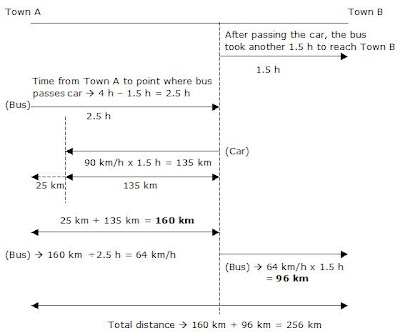### Rosyth Sch 2007 PSLE Math Prelim Q47

The perimeter of a rectangle to that of a square is in the ratio of 11:6. If the square has an area of 144 square m and the length and breadth of the rectangle are in the ratio of 6:5, find the length and breadth of the rectangle.

Solution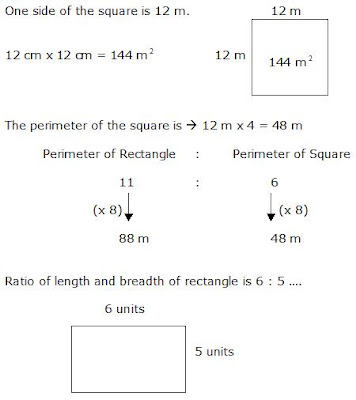Perimeter
(6 + 6 + 5 + 5) units ----- 88 m
22 units ----- 88 m
1 unit ----- 88m divided by 22 = 4 m

(Length) 6 units ----- 6 x 4 m = 24 m
(Breadth) 5 units ----- 5 x 4 m = 20 m

### Rosyth Sch 2007 PSLE Math Prelim Q46

The total cost of 28 textbooks and workbooks is \$784.
3/4 of the books are textbooks and the remaining books are workbooks. A workbook cost half as much as a textbook. Find the difference in the price of a textbook and a workbook.

Solution

Workbook ----- 1 unit
Textbook ------ 2 units

3/4 of the books are textbooks ----- 3/4 x 28 = 21

Workbooks ----- 28 – 21 = 7

21 textbooks + 7 workbooks ---- \$784

(21 x 2 units) + 7 units ----- \$784

42 units + 7 units ----- \$784

49 units ----- \$784

1 unit ------ \$784 divided 49 = \$16

## Friday, September 25, 2009

### Rosyth Sch 2007 PSLE Math Prelim Q45

The figure shows a cube with 3 painted parts A, B and C. These painted parts are of the same area and they are touching the midpoints of the sides of the cube. The total area of the painted parts is 54 square cm. Find the volume of the cube.3 painted parts on 3 faces of the cube ----- 54 square cm

1 painted part on 1 face of the cube
54 square cm divided by 3 = 18 square cm

Area of 1 triangle is 18 square cm divided by 2
= 9 square cm

For area of triangle to be 9 square cm, the base has to be 6 cm and height has to be 3 cm as worked out below
(1/2 x 6 cm x 3 cm = 9 square cm)

1 side of the cube is therefore 6 cm as seen from above diagram.

Volume of cube
(6 x 6 x 6) cubic cm = 216 cubic cm

### Rosyth Sch 2007 PSLE Math Prelim Q44

The figure is not drawn to scale. What fraction of the figure is the total area of the shaded regions A, B, C, D, E, F, G and H?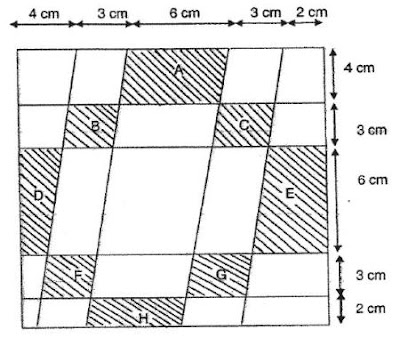Solution

The base of the whole figure is (4 + 3 + 6 + 3 + 2) cm = 18 cm

1st row from the top
Fig A has a base of 6 cm.

2nd row
The sum of the bases of Figs B and C is 6 cm.

3rd row
The sum of the bases of Figs D and E is 6 cm.

4th row
The sum of the bases of Figs F and G is 6 cm.

5th row
Fig H has a base of 6 cm.

The average length of the bases of all shaded figures is 6 cm.

The length of the base of the whole figure is 18 cm.

Therefore, the fraction of whole figure that is shaded is
6 cm divided by 18 cm = 1/3

Note that the above method can be used because the vertical columns also correspond to the 6 cm shaded out of the 18 cm total vertical length of the figure.

### Rosyth Sch 2007 PSLE Math Prelim Q43

The area of the figure ABCD is 48.5 square cm . Find the length of BE.Solution

Area of ACD
1/2 x 18 cm x 10.4 cm = 93.6 square cm

Area of ABC
93.6 square cm - 48.5 square cm = 45.1 square cm

Area of Triangle ABC ----- 1/2 x base x height
45.1 square cm = 1/2 x 18 cm x height

height = (45.1 sq cm x 2 ) divided by18 cm
= 5.01 cm ~ 5.0 cm (to the nearest tenth)

### Rosyth Sch 2007 PSLE Math Prelim Q41

The table below shows the rates at which a construction worker is being paid daily.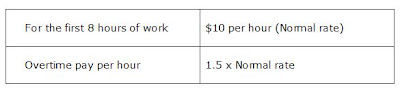How many hours must he work in a day to earn \$140?

Solution

First 8 hours of work ----- \$10 x 8 = \$80

To earn \$140, he needs another \$60.

Overtime rate is ----- \$10 per hour x 1.5 = \$15 per hour.

For overtime pay to be equal to \$60, he must work
\$60 divided by \$15 per hour = 4 hours

Total time he needs to work
8h + 4h = 12h

## Thursday, September 24, 2009

### Rosyth Sch 2007 PSLE Math Prelim Q40

Machine A can do a printing job in 2 hours. Machine B can do the same job in 3 hours. If both machines are used at the same time, how long would it take to complete half the printing job?

Solution

Since Machine A takes 2 hours and Machine B takes 3 hours, Machine A will be able to get more work done than Machine B, if both are used at the same time, in the ratio of 3:2 as shown below.(Machine A)
Whole job ----- 2 hours
3/5 of job ----- 3/5 x 2 hours = 6/5 hours

Time taken if both machines are used at the same time is 6/5 hours because while Machine A completes 3/5 of the job, Machine B completes the remaining 2/5 of the job.

Time taken for whole job done if both machines are used at same time is therefore 6/5 hours

Time taken for half the job done if both machines are used at the same time is
1/2 x 6/5 hours = 3/5 hour.

### Rosyth Sch 2007 PSLE Math Prelim Q39

Find the sum of the six marked angles in the diagram.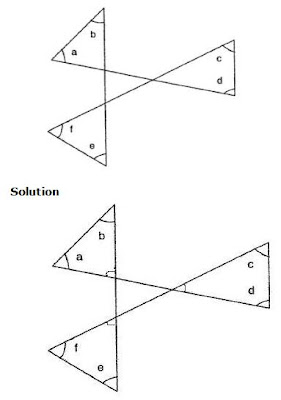The sum of the all the angles in the triangle in the centre is 180 degrees. The sum of all the 3 angles outside the centre triangle, in the figure above is therefore also 180 degrees (opposite angles).

The sum of all the six marked angles is hence,

Sum of marked angles in 1 triangle ----- 180 degrees
Sum of marked angles in 3 triangles ----- 180 degrees x 3 = 540 degrees
Sum of 6 marked angles ----- (540 – 180) degrees = 360 degrees

## Wednesday, September 23, 2009

### Rosyth Sch 2007 PSLE Math Prelim Q38

In the figure not drawn to scale, the ratio of the area of the bigger square to the smaller square is 8:4. If 25% of the larger square is shaded, what percentage of the whole figure is not shaded?Solution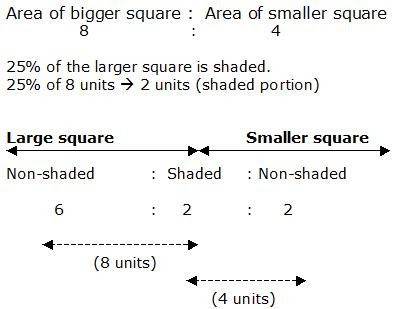Portion unshaded ----- 6 units + 2 units = 8 units
Total area ----- 6 units + 2 units + 2 units = 10 units

Percentage of figure that is not shaded
8/10 x 100% = 80%

### Rosyth Sch 2007 PSLE Math Prelim Q37

A tank with a square base of side 5 m was 1/3 full of water. When another 100 cubic meters of water was poured into the tank, it became 1/2 full. Find the capacity of the tank.

Solution

1/2 capacity – 1/3 capacity = 1/6 capacity

1/6 capacity ----- 100 cubic metres

6/6 capacity ----- 6 x 100 cubic m = 600 cubic m

### Rosyth Sch 2007 PSLE Math Prelim Q36

The average weight of 5 boys is 8w kg. When 2 more children whose weights are 11w kg and 12 w kg respectively joined the group, what is the average weight of the boys now?

Solution

Average weight of 5 boys ----- 8w kg

Total weight of 5 boys ----- 8w kg x 5 = 40w kg

Total weight of 7 boys
(40w + 11w + 12w) kg = 63w kg

Average weight of 7 boys
63w kg divided by 7 = 9w kg

## Tuesday, September 22, 2009

Excel Eduservice would like to thank everyone for supporting this website. We are pleased this site has helped many P6 students, parents and tutors the last 2 years.

Free Maths Worked Solutions and PLSE Aggregate Score Calculator

There is a NEW free maths worked solution (Nanyang Pri P6 Prelim 2007) in the free section above.

At Excel Eduservice, we work to serve you better.

You're in good hands when you're with Excel!

### Raffles Girls Pri Sch 2007 PSLE Math Prelim Q48

Valley Department Store sold a dress for \$585. This was 17% more than the price of a similar dress in Wiki Department Store. During a sale, both stores offered same percentage discount on the dress. Christine bought the dress in Wiki Department Store and found that she paid \$68 less than the discounted price in Valley Department Store.

(a) Find the price of the dress in Wiki Department Store before the sale.
(b) What is the percentage discount given during the sale?

Solution

(a)

Valley ----- 100% + 17% = \$585
117% ----- \$585
1% ----- \$585 divided by 117 = \$5

(Wiki) 100% ----- 100 x \$5 = \$500

(b)
Before discount\$85 is the difference in price between Valley and Wiki before the discount. After the discount, the difference was \$68.

\$85 - \$68 = \$17
Discount ----- (\$17/\$85) x 100% = 20%

### Raffles Girls Pri Sch 2007 PSLE Math Prelim Q47

Melissa spent 3/8 of her money on 3 pencils and 8 pens, and 4/5 of the remainder on 15 markers. Each pencil cost 2/3 as much as a pen. Each marker cost \$0.20 more than a pencil. What is the cost of a pen?

Solution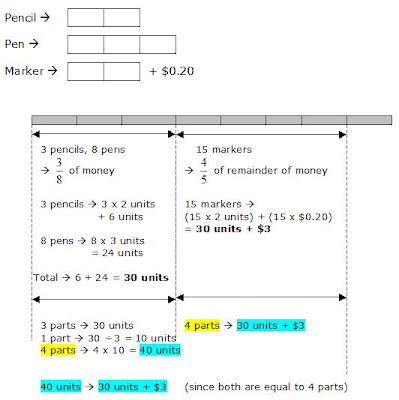40 units – 30 units ----- \$3
10 units ----- \$3
1 unit ----- \$3 divided by 10 = \$0.30

(Pen) 3 units ----- \$0.30 x 3 = \$0.90

## Monday, September 21, 2009

### Raffles Girls Pri Sch 2007 PSLE Math Prelim Q46

At first, the ratio of the number of marbles received by John and Peter was 4:7. The ratio of the number of marbles received by Peter and Sam was 9:5. Then, John gave 1/12 of his marbles to Sam, and Peter gave 1/9 of his marbles to Sam. As a result, Sam had 135 marbles in the end.

(a) Find the ratio of the number of John’s marbles to the number of Sam’s marbles at first.

(b) Find the total number of marbles received by the 3 boys.

Solution45 units ----- 135 (marbles)
1 unit ----- 135 divided by 45 = 3
134 units ----- 134 x 3 = 402

## Thursday, September 17, 2009

### Raffles Girls Pri Sch 2007 PSLE Math Prelim Q45

The figure below shows a rectangle PQRS. The lines are extended from point P, Q, R and S and they meet at point Y. The length of QR is 20 cm and the length of XY is 4 cm. Given that QS is a straight line, the area of Triangle PQY is 72 square cm and the area of Triangle SRY is 84 square cm, find the shaded area of Triangle QSY.Solution

Area of Triangle PQY + Area of Triangle SRY
72 sq cm + 84 sq cm = 156 square cm

Area covered by Triangles PQY and SRY is also 1/2 of area of Rectangle PQRS. This also means that Area of Triangle PQS is also 156 sq cm.

Area of Triangle PSY = 1/2 x 20 cm x 4 cm = 40 sq cm

Area of Triangle PQY = 72 sq cm

Area of PQS – Area of PQY – Area of PSY
=156 sq cm - 72 sq cm - 40 sq cm= 44 square cm

### Raffles Girls Pri Sch 2007 PSLE Math Prelim Q44

A group of pupils sat for two tests, test A and test B. The number of pupils who failed test A was 1/7 of those who passed test A. Given that there were 20 pupils who failed test A,

(a) How may pupils passed test A?
(b) The number of pupils who failed test B was 1/4 of those who failed test A. What fraction of the pupils passed test B?

Solution(a)
1 unit ----- 20
7 units ----- 20 x 7 = 140

(b)
Failed Test A ----- 20
Failed Test B ----- 1/4 x 20 = 5

(Total number of pupils)
8 units ----- 8 x 20 = 160

Fraction of pupils who failed Test B
5/160 = 1/32

Fraction of pupils who passed Test B
1 – 1/32 = 31/32

## Wednesday, September 16, 2009

### Raffles Girls Pri Sch 2007 PSLE Math Prelim Q43

There are some red and green beads in a container. If 75 more green beads are put into the container, the percentage of red beads will decrease from 30% to 20%. How many red beads are in the container?

Solution12 units ----- 7 units + 75
5 units ----- 75
1 unit ----- 75 divided by 5 = 15

Red at first
3 units ----- 3 x 15 = 45

## Tuesday, September 15, 2009

### Raffles Girls Pri Sch 2007 PSLE Math Prelim Q41

Jane started driving at 9.30 am from Town A to Town B. At 11.30 am, Jane had covered only 2/5 of the distance. She had to cover another 144 km before she reached Town B.

(a) What was the distance between Town A and Town B?

(b) If Jane were to travel at an average speed of 72km/h after 11.30 am, at what time would she reach Town B?

Solution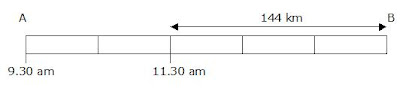(a)
3 units ----- 144 km
1 unit ----- 144 km divided by 3 = 48 km
5 units ----- 5 x 48 km = 240 km

(b)
Time = 144km divided by 72 km/h = 2 h
2 hours after 11.30 am is 1.30 pm or 1330 h.

## Monday, September 14, 2009

### Raffles Girls Pri Sch 2007 PSLE Math Prelim Q40

A rectangular tank with 5 identical solid metal cubes was filled to the brim with water. Water was drained out of the tank through a tap at a rate of 2 litres per minute. It took 18 minutes for the water level to drop to the height of the metal cubes. Find the volume of the 5 metal cubes.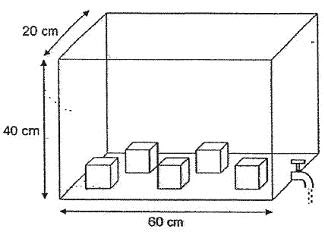Solution

Volume of whole tank
60 cm x 20 cm x 40 cm = 48 000 cubic cm or 48 litres

1 min ----- 2 litres

18 min ----- 2 litres x 18 = 36 litres

Volume of tank filled with water + volume of cubes after 18 min
48 litres – 36 litres = 12 litres or 12 000 cm

Height of cubes is therefore,
Height ------ Volume divided by base area
= 12 000 cubic cm divided by (60 cm x 20 cm) = 10 cm

Height of cube ------ 10 cm
Volume of 1 cube ----- 10 cm x 10 cm x 10 cm = 1000 cubic cm
Volume of 5 cubes ----- 1000 cubic cm x 5 = 5000 cubic cm

## Wednesday, September 09, 2009

### Anglo Chinese School 2007 PSLE Math Prelim Q48

Mr Goh was travelling from Town X to Town Y. After completing 2/7 of the journey, he passed by Mr Lee travelling the same direction. Mr Lee was travelling at an average speed of 60 km/h. Mr Goh reached his destination 3 hours later, while Mr Lee was still 45 km away from Town Y.

(a)Find the distance between the two towns.
(b)If Mr Lee left Town x at 11.30 am, what time would he arrive at Town Y?

Solution(a)
(Goh) 5/7 of journey ----- 225 km
1/7 of journey ----- 225 km 5 = 45 km
(Whole journey) 7/7 ----- 45 km x 7 = 315 km

(b)
Lee’s time ----- distance divided by speed
315 km divided by 60 km/h
= 5.25 hours or 5 hours 15 min

Since Lee left at 11.30 am, he would arrive at 4.45 pm.

## Tuesday, September 08, 2009

### Anglo Chinese School 2007 PSLE Math Prelim Q47

In an auditorium, the ratio of the number of competitors to the number of non-competitors is 8:5. The ratio of the number of male competitors to the number of female competitors is 7:4. Given that 3/5 of the non-competitors are males and there are 32 female competitors, how many males and females are there in the auditorium?

SolutionMales ----- 56 + 33 = 89
Females ----- 32 + 22 = 54

### Anglo Chinese School 2007 PSLE Math Prelim Q46

A rectangular container measuring 45 cm by 10 cm by 20 cm is empty at first. Water flows from Tap X at a rate of 1.3 litres per minute and from Tap Y at a rate of 1.2 litres per minute. How long does it take to completely fill up the container if both taps are turned on at the same time? Give your answer in minutes and seconds.

Solution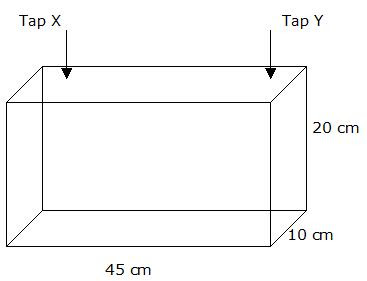Volume of tank ----- 45 cm x 10 cm x 20 cm
= 9000 square cm or 9 litres

1 min ----- 1.3 litres/min + 1.2 litres/min = 2.5 litres/min

9 litres divided 2.5 litres/min = 3.6 min or 3 min 36 seconds

## Monday, September 07, 2009

### Anglo Chinese School 2007 PSLE Math Prelim Q45

The figure is made up of three circles and two semi-circles. O is the center of the circle. AB is 42 cm. Find the total area of the shaded part.Area of 1 square ----- (10.5 x 10.5)square cm = 110.25 square cm

Area of 2 squares ----- 2 x 110.5 square cm = 220.5 square cm

Area of shaded area is ---- Area of whole figure – area of 1 small circle – area of 4 small quadrants – area of 2 squares

(1386 - 346.5 - 346.5 - 220.5) square cm = 472.5 square cm

## Sunday, September 06, 2009

### Anglo Chinese School 2007 PSLE Math Prelim Q44

Marvin bought a box of fruits. 30% of the fruits are apples and the rest are oranges. He realized that half of the apples were rotten and threw them away. He then bought some oranges and the number of oranges increased by 40%. After that, he found that there were 52 more fruits in the box. How many fruits were there in the box at first?

Solution

At first
Apples ----- 30%
Oranges ----- 70%

Threw half apples away, bought 40% more oranges,
Apples ----- 1/2 x 30% = 15%
Oranges ----- 70% + (40/100 x 70%) = 98%

Total ----- 15% + 98% = 113%

113% - 100% = 13% (increase in number of fruits)
13% ----- 52 fruits
1 % ----- 52% divided by 13 = 4

Number of fruits at first,
100% ----- 4 x 100 = 400

### Anglo Chinese School 2007 PSLE Math Prelim Q43

Derrick had 2/3 as many stickers as Benedict. After Derrick bought another 8 stickers and Benedict lost 5 stickers, Derrick now has 4/5 as many stickers as Benedict. Find the number of stickers Derrick and Benedict had at first.

Solution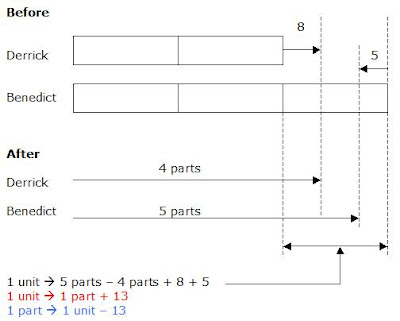(Derrick after)
4 parts ----- 2 units + 8
2 parts ----- 1 unit + 4
2 parts ----- 1 part + 13 + 4
2 parts – 1 part ----- 17
1 part ----- 17

1 unit – 13 ----- 17
1 unit ----- 17 + 13 = 30
5 units ----- 30 x 5 = 150

### Anglo Chinese School 2007 PSLE Math Prelim Q41

Alex has \$1.50 more money than Betty and three times as much money as Colin. The 3 of them have \$9.70 altogether. How much does Colin have?

Solution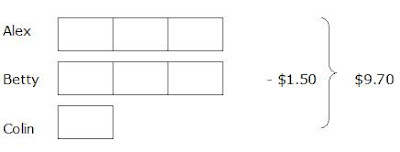7 units - \$1.50 ----- \$9.70

7 units ----- \$9.70 + \$1.50 = \$11.20

1 unit ----- \$11.20 divided by 7 = \$1.60

### Anglo Chinese School 2007 PSLE Math Prelim Q40

Fara started collecting stamps in January. In each month from February to May, she collected 30 stamps more than the month before. She saved a total of 750 stamps from January to May. How many stamps did she collect in January?

Solution5 units + 10 groups of 30 ---- 750

5 units + 300 ---- 750

5 units ---- 750 – 300 = 450

1 unit ---- 450 divided by 5 = 90

## Saturday, September 05, 2009

### Anglo Chinese School 2007 PSLE Math Prelim Q38

In the figure not drawn to scale, ABCD is a square. BDE and BCF are equilateral triangles. What is Angle FGE?Solution

Angle BCF ---- 60º (Triangle BCF is an equilateral triangle)

Angle CBD ---- 45º

Angle CBG ---- 60º - 45º = 15º

Angle BGC ---- 180º - 60º - 15º = 105º (sum of angles in a triangle)

Angle FGE ---- 105º (opposite angles)

## Wednesday, September 02, 2009

### Singapore Chinese Girls Sch 2008 PSLE Math Prelim Q48

At a farm, 40% of the animals are cows, 90% of the remainder are sheep and the rest are ducks. There are 56 more sheep than cows. After some cows died, 20% of the remaining animals at the farm are cows. How many cows are there left at the farm?

Solution

Cows ---- 40%
Remainder (sheep + ducks) ---- 100% - 40% = 60%

Sheep ---- 90% (remainder) x 60% = 54%

Sheep more than cows ---- 56
(Sheep) – (Cows) ---- 56
54% - 40% ---- 56
14% ---- 56
1% ---- 56 divided by 14 = 4

(Total) 100% ---- 100 x 4 = 400
(Sheep + ducks) ---- 60%
60% ---- 60 x 4 = 240

After some cows died 80% were sheep and ducks
80% ---- 240
1% ---- 240 divided by 80 = 3

(Cows left) 100% - 80% = 20%
20% ---- 20 x 3 = 60

Answer: There were 60 cows left.